Gaurav Singhal

# Artistic Neural Style Transfer with TensorFlow 2.0, Part 2: Implementation

• Jun 3, 2020
• 4,237 Views
• Jun 3, 2020
• 4,237 Views
Data
Data Analytics
Machine Learning
Tensorflow

## Introduction

This is the second guide in a two-part series on artistic neural style transfer. Part 1 walked through separating the convolution layer for style and content images to extract their respective features. When the loss function is tuned, it combines these features to generate a styled image. This guide, Part 2, will go deeper into style loss and content loss.

Usually, in deep learning, we have only one loss function. However, in neural style transfer, we are generating a new image from two images, so we need more loss functions to generate a new image. We will discuss various loss functions such as content loss, style loss, and variation loss.

There are many approaches to mathematical notation. The equations in this guide are taken from Gatsy et al. (some notations might differ).

Below is a simple representation of how the new image will be generated from the content and style images.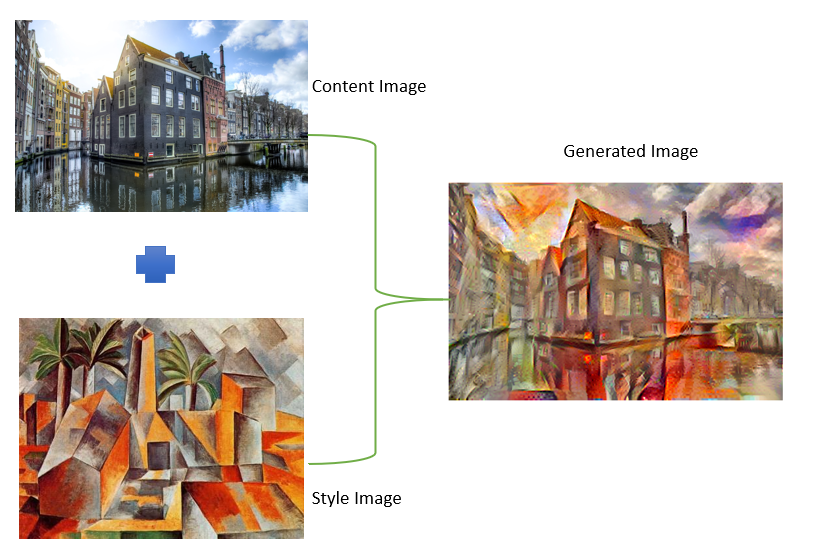## Content Loss

This function helps to check how similar the generated image is to the content image. It gives the measure of how far (different) are the features of the content image and target image. The Euclidean distance is calculated. It is defined as follows: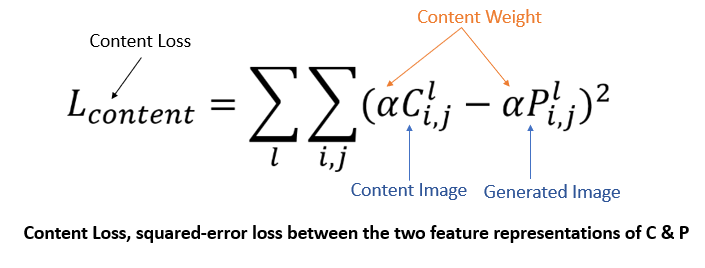## Style Loss

Style Loss measures how different the generated image, in terms of style features, is from your style image. But it's not as straightforward as content loss. The style representation of an image is given by Gram Matrix.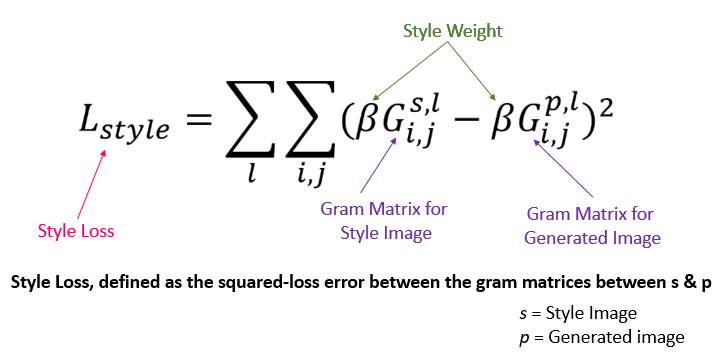## Gram Matrix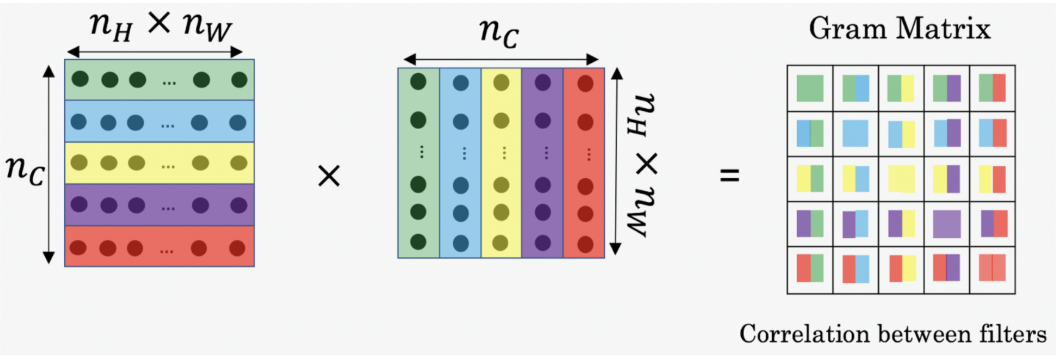Gram Matrix is only concerned with whether the stylish features are present in image weights, textures, and shapes. Hence, it is the best choice. The Gram Matrix G is the set of vectors in a matrix of dot products. For a particular layer, the diagonal elements of the matrix will find how active the filter is. An active filter will help the model find wether it contains more horizontal lines, vertical lines, or textures.

To get the results, the matrix is multiplied by its transposed matrix.

Its equation is as follows: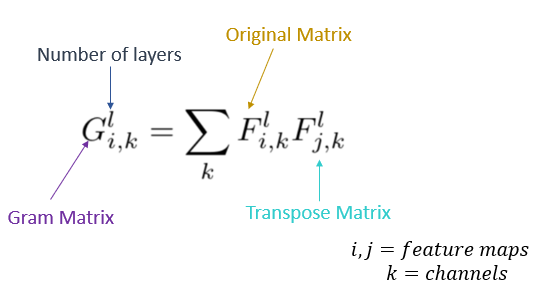The GM helps you find how similar Fik is to Fjk. If the dot product is large, they are highly similar.

Finally, total loss will minimize the weighted average.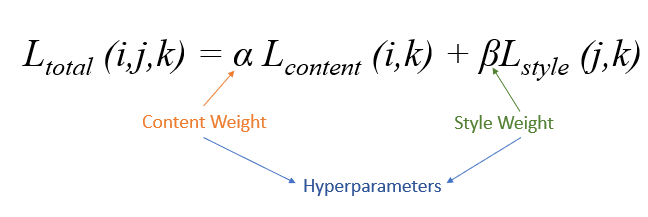### Variation Loss

Variation loss was introduced to avoid highly noisy outputs and overly pixelated results. The main purpose of variation loss is to maintain smoothness and spatial continuity.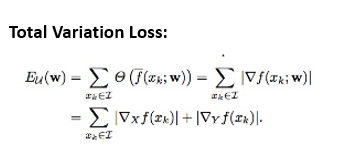The change in the combination of images minimizes the loss so that you can have an image combination of both the Picasso painting and the input image. To make the losses bit smaller, an optimization algorithm is used. The first order algorithm uses a gradient to minimize the loss function, famously known as gradient descent. The Adam optimization shows faster results in style transfer.

## Code Implementation

In this section, we will implement the code to generate a Gram Matrix from the input image tensor and the model that will generate the image.

GM can be implemented concisely using the `tf.linalg.einsum` function:

``````1def gram_matrix(input_tensor):
2  result = tf.linalg.einsum('bijc,bijd->bcd', input_tensor, input_tensor)
3  input_shape = tf.shape(input_tensor)
4  num_locations = tf.cast(input_shape*input_shape, tf.float32)
5  return result/(num_locations)``````
python

Build a model that returns the style and content tensors.

``````1class StyleContentModel(tf.keras.models.Model):
2  def __init__(self, style_layers, content_layers):
3    super(StyleContentModel, self).__init__()
4    self.vgg =  vgg_layers(style_layers + content_layers)
5    self.style_layers = style_layers
6    self.content_layers = content_layers
7    self.num_style_layers = len(style_layers)
8    self.vgg.trainable = False
9
10  def call(self, inputs):
11    "Expects float input in [0,1]"
12    inputs = inputs*255.0
13    preprocessed_input = tf.keras.applications.vgg19.preprocess_input(inputs)
14    outputs = self.vgg(preprocessed_input)
15    style_outputs, content_outputs = (outputs[:self.num_style_layers],
16                                      outputs[self.num_style_layers:])
17
18    style_outputs = [gram_matrix(style_output)
19                     for style_output in style_outputs]
20
21    content_dict = {content_name:value
22                    for content_name, value
23                    in zip(self.content_layers, content_outputs)}
24
25    style_dict = {style_name:value
26                  for style_name, value
27                  in zip(self.style_layers, style_outputs)}
28
29    return {'content':content_dict, 'style':style_dict}``````
python

When called on an image, this model returns the gram matrix (style) of the `style_layers` and content of the `content_layers`:

``````1extractor = StyleContentModel(style_layers, content_layers)
2
3results = extractor(tf.constant(content_image))
4
5print('Styles:')
6for name, output in sorted(results['style'].items()):
7  print("  ", name)
8  print("    shape: ", output.numpy().shape)
9  print("    min: ", output.numpy().min())
10  print("    max: ", output.numpy().max())
11  print("    mean: ", output.numpy().mean())
12  print()
13
14print("Contents:")
15for name, output in sorted(results['content'].items()):
16  print("  ", name)
17  print("    shape: ", output.numpy().shape)
18  print("    min: ", output.numpy().min())
19  print("    max: ", output.numpy().max())
20  print("    mean: ", output.numpy().mean())``````
python``````1style_targets = extractor(style_image)['style']
2content_targets = extractor(content_image)['content']``````
python

The `tf.variables` are used to assign biases and weights throughout the training session. These weights are then used for optimization. They are initialized with the content image.

Note: `tf.variable` and the content image are the same size.

``1image = tf.Variable(content_image)``
python

Since this is a float image, define a function to keep the pixel values between 0 and 1:

``````1def clip_0_1(image):
2  return tf.clip_by_value(image, clip_value_min=0.0, clip_value_max=1.0)``````
python

Set the variables for the Adam optimizer.

``1opt = tf.optimizers.Adam(learning_rate=0.02, beta_1=0.99, epsilon=1e-1)``
python

Get the total loss use the weighted combination of style and content losses.

``````1style_weight=1e-2
2content_weight=1e4``````
python

Now comes the main part: loss function!

``````1def style_content_loss(outputs):
2    style_outputs = outputs['style']
3    content_outputs = outputs['content']
5                           for name in style_outputs.keys()])
6    style_loss *= style_weight / num_style_layers
7
9                             for name in content_outputs.keys()])
10    content_loss *= content_weight / num_content_layers
11    loss = style_loss + content_loss
12    return loss``````
python

The `tf.function` will speed up the operation. Defining `train_step` will send the gradient to the optimizer. `tf.GradientTape()` calculates the gradients of the function based on its composition automatically.

``````1@tf.function()
2def train_step(image):
4    outputs = extractor(image)
5    loss = style_content_loss(outputs)
6
9  image.assign(clip_0_1(image))``````
python

Now run a few steps to test:

``````1train_step(image)
2train_step(image)
3train_step(image)
4tensor_to_image(image)``````
python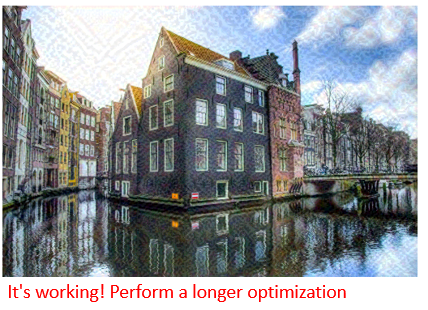``````1import time
2start = time.time()
3
4epochs = 10
5steps_per_epoch = 100
6
7step = 0
8for n in range(epochs):
9  for m in range(steps_per_epoch):
10    step += 1
11    train_step(image)
12    print(".", end='')
13  display.clear_output(wait=True)
14  display.display(tensor_to_image(image))
15  print("Train step: {}".format(step))
16
17end = time.time()
18print("Total time: {:.1f}".format(end-start))``````
python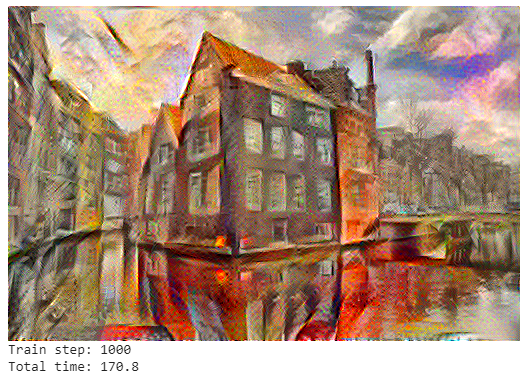Now add total variation loss to reduce the high frequency artifacts. Apply high frequency explicit regularization term on the high frequency components of the image. The difference between the neighboring pixels is shown below:

``````1def high_pass_x_y(image):
2  x_var = image[:,:,1:,:] - image[:,:,:-1,:]
3  y_var = image[:,1:,:,:] - image[:,:-1,:,:]
4
5  return x_var, y_var``````
python

The comparison of horizontal (width) and vertical (height) high-frequency components (edge-detection) for content of a styled image is shown below.

``````1x_deltas, y_deltas = high_pass_x_y(content_image)
2
3plt.figure(figsize=(14,10))
4plt.subplot(2,2,1)
5imshow(clip_0_1(2*y_deltas+0.5), "Horizontal Deltas: Original")
6
7plt.subplot(2,2,2)
8imshow(clip_0_1(2*x_deltas+0.5), "Vertical Deltas: Original")
9
10x_deltas, y_deltas = high_pass_x_y(image)
11
12plt.subplot(2,2,3)
13imshow(clip_0_1(2*y_deltas+0.5), "Horizontal Deltas: Styled")
14
15plt.subplot(2,2,4)
16imshow(clip_0_1(2*x_deltas+0.5), "Vertical Deltas: Styled")``````
python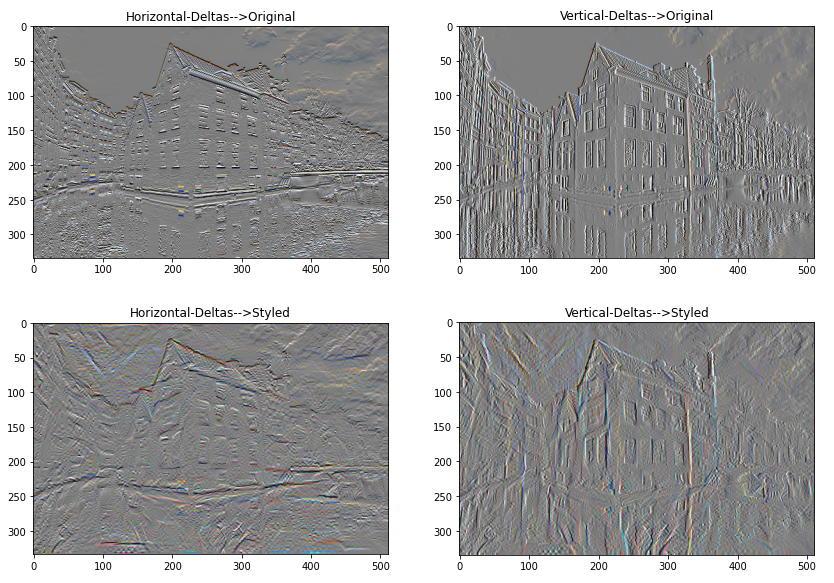This shows how the high-frequency components have increased.

You can get similar output from the Sobel edge detector, for example:

``````1plt.figure(figsize=(14,10))
2
3sobel = tf.image.sobel_edges(content_image)
4plt.subplot(1,2,1)
5imshow(clip_0_1(sobel[...,0]/4+0.5), "Horizontal Sobel-edges")
6plt.subplot(1,2,2)
7imshow(clip_0_1(sobel[...,1]/4+0.5), "Vertical Sobel-edges")``````
python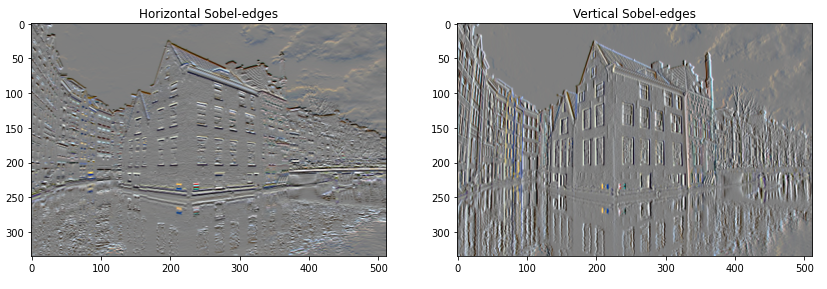Optimize the squared value by finding the rate of change of edges with `high_pass_x_y`.

``````1def total_variation_loss(image):
2  x_deltas, y_deltas = high_pass_x_y(image)
3  return tf.reduce_sum(tf.abs(x_deltas)) + tf.reduce_sum(tf.abs(y_deltas))``````
python
``1total_variation_loss(image).numpy()``
python

Output: 89581.1

``1tf.image.total_variation(image).numpy()``
python
``1array([89581.1], dtype=float32)``

Re-run the optimization and adjust total variation weight.

``1total_variation_weight=30``
python
``````1@tf.function()
2def train_step(image):
4    outputs = extractor(image)
5    loss = style_content_loss(outputs)
6    loss += total_variation_weight*tf.image.total_variation(image)
7
10  image.assign(clip_0_1(image))``````
python
``1image = tf.Variable(content_image)``
python

Call the overall method. Notice the change in patterns after every 100 epochs.

``````1import time
2start = time.time()
3
4epochs = 10
5steps_per_epoch = 100
6
7step = 0
8for n in range(epochs):
9  for m in range(steps_per_epoch):
10    step += 1
11    train_step(image)
12    print(".", end='')
13  display.clear_output(wait=True)
14  display.display(tensor_to_image(image))
15  print("Train step: {}".format(step))
16
17end = time.time()
18print("Total time: {:.1f}".format(end-start))``````
python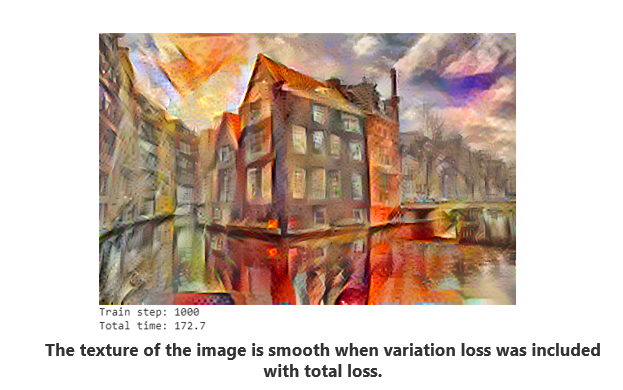Save the results.

## Conclusion

Congratulations! You are the owner of unique, amazing digital art. You can now play with different images and paintings to adjust the weights of style and content features and see the changes. I also recommend reading this blog to gain in-depth knowledge of gradient descent.

This guide also contains many mathematical equations, and I recommend reading the paper mentioned above to understand their purpose. Knowing the purpose will help you modify them if required.

This whole implementation is done in Tensorflow 2.0. When you run it, you'll notice that it is much slower even on GPUs. In a future guide, we will look at how to implement a shorter and faster version of the same functionality in PyTorch.